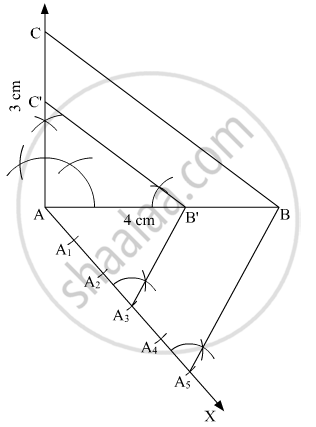Share

# Draw a Right Triangle in Which the Sides (Other than the Hypotenuse) Are of Lengths 4 Cm and 3 Cm. Now Construct Another Triangle Whose Sides Are 35 Times the Corresponding Sides of the Given Triangle. - CBSE Class 10 - Mathematics

#### Question

Draw a right triangle in which the sides (other than the hypotenuse) are of lengths 4 cm and 3 cm. Now construct another triangle whose sides are 3/5 times the corresponding sides of the given triangle.

#### Solution

Construct a right triangle of sides AB = 4 cm, AC = 3 cm and ∠A = 90° and then a triangle similar to it whose sides are (3/5)^"th" of the corresponding sides of ΔABC

We follow the following steps to construct the givenStep of construction

Step: I- First of all we draw a line segment AB = 4 cm.

Step: II- With A as centre and draw an angle ∠A = 90°.

Step: III- With A as centre and radius AC = 3 cm.

Step: IV-Join BC to obtain right ΔABC.

Step: V- Below AB, makes an acute angle ∠BAX.

Step: VI- Along AX, mark off five points A1, A2, A3, A4 and A5 such that =A4A5.

Step: VII- Join A5B.

Step: VIII -Since we have to construct a triangle each of whose sides is (3/5)th of the corresponding sides of right ΔABC

So, we draw a line on AX from point which is A3B ∥ A5B and meeting AB at B’.

Step: IX- From B’ point draw B'C' || BCand meeting AC at C’

Thus, ΔABC is the required triangle, each of whose sides is (3/5)th of the corresponding sides of ΔABC.

Is there an error in this question or solution?

#### APPEARS IN

Solution Draw a Right Triangle in Which the Sides (Other than the Hypotenuse) Are of Lengths 4 Cm and 3 Cm. Now Construct Another Triangle Whose Sides Are 35 Times the Corresponding Sides of the Given Triangle. Concept: Division of a Line Segment.
S